# Do Pro Golfers Feed off of their playing partners? Part 2 (I was wrong!)

FeedoffEffect2-Copy1

In part one of this post (here), I explored whether there is a statistically significant correlation between the performance of players playing in the same grouping on the PGA Tour. I found evidence that there is a statistically significant correlation by comparing the within group variation of performance to the between group variation with ANOVA and the Kruskal-Wallis H-test. I also ran a non-parametric permutation test which also provided strong evidence for correlation of performance within a group.

In this part, I am going to go back over my assumptions and make my analysis more robust by controlling for a possible lurking variable. Then, if the evidence is still strong that there is an effect, I will explore some of the questions that I posed at the beginning of part 1: does this effect exist especially at certain tournaments? On certain courses? For certain players? During certain times? Under certain circumstances?

I will start by fastforwarding through all the data cleaning and validation steps that I performed in part one. I have made a script which performs all the transformations (motivated in part 1) and writes out a clean dataframe.

In :
import pandas as pd
pd.options.display.max_columns = 999
import numpy as np
import warnings
import matplotlib.pyplot as plt
%matplotlib inline

In :
## read in data and take a look at it


Out:
Tournament_Year Permanent_Tournament_# Course_# Player_Number Player_Name Round_Number Event_Name Course_Name Tee_Time Round_Score End_of_Round_Finish_Pos._(text) Hole_Started_On Field_Ave Strokes_Gained Exp_StrokesGained Net_Result
0 2003 16 656 1320 Estes, Bob 1 Mercedes Championships Plantation Course at Kapalua 11:50 66 T6 1.0 69.361111 3.361111 0.0 3.361111
1 2003 16 656 1361 Forsman, Dan 1 Mercedes Championships Plantation Course at Kapalua 11:00 69 T14 1.0 69.361111 0.361111 0.0 0.361111
2 2003 16 656 1761 Mattiace, Len 1 Mercedes Championships Plantation Course at Kapalua 13:00 75 36 1.0 69.361111 -5.638889 0.0 -5.638889

To remind people what the last 4 columns are:

• Field_Ave is the average for the field during that particular day
• Strokes_Gained is Field_Ave - Round_Score. It is a raw measure of performance for the round
• Exp_StrokesGained is an exponentially-weighted moving average of the player's past Strokes_Gained. The halflife parameters was tuned to be most predictive.
• Net_Result is Strokes_Gained - Exp_StrokesGained. It is a measure of performance against personalized expected performance.

Exp_StrokesGained is 0 for the first round since we had no past experience with which to produce an expectation (the data are ordered chronologically).

In :
## data ends with the last tournament of 2016 - the tour championship
round_data.tail(3)

Out:
Tournament_Year Permanent_Tournament_# Course_# Player_Number Player_Name Round_Number Event_Name Course_Name Tee_Time Round_Score End_of_Round_Finish_Pos._(text) Hole_Started_On Field_Ave Strokes_Gained Exp_StrokesGained Net_Result
252770 2016 60 688 34360 Reed, Patrick 4 TOUR Championship East Lake GC 12:25 70 T24 1.0 68.0 -2.0 1.050367 -3.050367
252771 2016 60 688 37455 Kim, Si Woo 4 TOUR Championship East Lake GC 12:15 65 T10 1.0 68.0 3.0 0.747430 2.252570
252772 2016 60 688 40026 Berger, Daniel 4 TOUR Championship East Lake GC 12:45 68 T15 1.0 68.0 0.0 0.749491 -0.749491
In :
## how many rounds in the data?
len(round_data)

Out:
252773

In part 1, the tests for statistical significance that I ran all assumed the the samples - the results in each group - were independent. I realized that if I did not control for skill of each player, the samples would not be independent because of the way the groupings are done - players that have done well in previous rounds are grouped together. This makes the samples of unadjusted performance not independent. To control for skill, I computed an expected performance for each golfer using his past performance and the new result variable I considered was his actual result minus his expected result.

An analogy is if we looked at whether performance on a standardized test was linked to which teacher you had at a particular school. For this we'd consider the samples to be each teacher's students and we'd look at whether the within group variation was less than what you'd expect from random chance. But what if the classes were formed by considering students' aptitude? Then the samples would no longer be independent. The correct for this, you could form a prediction of each student's performance on the test using data from before the classes were formed and use that prediction to control for inherent aptitude. This is what I did for the golfers.

I've since realized that there might be an additional lurking variable that could invalidate the assumption of independence of the samples - the difficulty of the conditions varying throughout a particular round. Since all the players in a group play under the same conditions, it stands to reason that the players' performances would vary less than you'd expect according to chance (when compared against all other groups on the same day) as the difficult of the conditions can vary throughout the day. For example, the afternoon tends to be windier than the mornings which can make the conditions more difficuly in the afternoon.

To continue the earlier analogy, let's say the students sit next to their classmates in a big auditorium. Let's say there is a distraction at one end of the room which continues thoughout the test. This would make the conditions more difficult for the classes who are sitting closest to the distraction. If the distraction impacted performance especially for certain classes (those sitting closest), the within group variation would be less than you'd expect according to chance. In other words, the samples are no longer independent because of a lurking variable.

I would like to control for this potential lurking variable and see if the effect that I found in part 1 holds up. It would be great to have really detailed weather data for each of these rounds, but I am not sure of a reliable, granular source. Instead I will use the data from within each round and use Tee_Time to fit a model that predicts the Net_Result variable. I'll then subtract the prediction from each model from the Net_Result, to obtain an outcome that I believe will be more independent than Net_Result.

Since the relationship between Tee_Time and Net_Result within each round might be non-linear, I will use a natural spline to fit the model, fitting the degrees of freedom using cross-validation.

In :
## Define a class for a Natural Spline
## https://web.stanford.edu/~hastie/Papers/ESLII.pdf#page=163
class NaturalSpline():
def __init__(self,n_folds=6):
self.n_folds = n_folds

def get_mat(self,x,df):
notches = np.percentile(x,np.linspace(0,100,df+2)[1:-1])
X = np.column_stack(([1.0]*len(x),x))
def d(x,e_k,e_K):
def pos(x,e):
x_ = np.copy(x)
x_[x<=e] = 0.0
x_[x>e] = (x_[x>e]-e)**3
return x_
return (pos(np.copy(x),e_k) -
pos(np.copy(x),e_K))/(e_K-e_k)
for i in range(1,len(notches)-1):
X = np.column_stack((X,d(np.copy(x),notches[i],
notches[-1]) -
d(np.copy(x),notches[i-1],
notches[-1])))
return X

def score(self,X,y,n_iterations=50):
from sklearn.model_selection import KFold
from sklearn.linear_model import LinearRegression
model = LinearRegression(fit_intercept=False)
scores = []
for seed in np.random.randint(0,10000,size=n_iterations):
cv = KFold(n_splits=self.n_folds,shuffle=True,
random_state=seed)
for train,test in cv.split(X,y):
model.fit(X[train],y[train])
scores.append(model.score(X[test],y[test]))
return np.mean(scores)

def fit(self,x,y,dfs=range(3,10)):
scores = []
num_unique_x = len(np.unique(x))
if num_unique_x<min(dfs):
raise ValueError('x needs to have at least ' +\
'%d unique values' % (min(dfs),))
if len(x)<self.n_folds:
raise ValueError('x needs to have length of at ' +\
'least %d' % (self.n_folds,))
for df in dfs:
if df<=num_unique_x:
X = self.get_mat(x,df)
scores.append(self.score(X,y))
self.scores = scores
df = dfs[np.argmax(scores)]
self.df = df
X = self.get_mat(x,df)
from sklearn.linear_model import LinearRegression
model = LinearRegression(fit_intercept=False)
model.fit(X,y)
self.model = model

def predict(self,x):
return self.model.predict(self.get_mat(x,self.df))


Let's try this for one day's worth of rounds to get a sense of what it does.

In :
day = round_data[(round_data.Tournament_Year==2003) &
(round_data['Permanent_Tournament_#']==1) &
(round_data['Course_#']==101) &
(round_data.Round_Number==3)]

In :
## helper function to represent the tee times as seconds
## since first tee time
def get_seconds(x):
x = pd.to_timedelta(x + ':00').apply(lambda x: x.seconds)
return x - x.min()

In :
## get x and y
x = get_seconds(day.Tee_Time).values
y = day.Net_Result.values

## make a plot
plt.scatter(x,y);
plt.xlabel('Seconds Since First Tee Time');
plt.ylabel('Net_Result');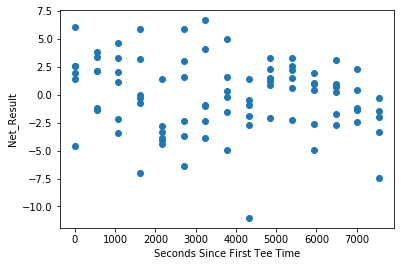In :
## define NaturalSpline object, fit it
ns = NaturalSpline()
ns.fit(x,y)

In :
## number of degrees of freedom chosen using cv
ns.df

Out:
5
In :
## predict x values
y_ = ns.predict(x)

## make a plot
plt.scatter(x,y);
plt.plot(x[np.argsort(x)],y_[np.argsort(x)],c='red');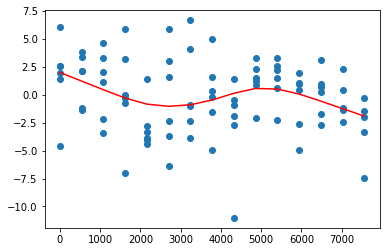In :
## how many strokes easier does the model say teeing off
## first was than teeing off last on this day?
y_[np.argsort(x)] - y_[np.argsort(x)[-1]]

Out:
3.9081229599840031

So this model thinks teeing off last was almost two strokes harder than teeing off first. That is actually a lot.

In :
## just for fun do an animation showing a bunch of the same sort
## of plots for different days
day_identifiers = ['Tournament_Year','Permanent_Tournament_#',
'Course_#','Round_Number']
xs, ys, y_s = [], [], []
for tup in round_data[day_identifiers].drop_duplicates().values[:50]:
year,tourn_num,course_num,round_num = tup
mask = (round_data.Tournament_Year==year) & \
(round_data['Permanent_Tournament_#']==tourn_num) & \
(round_data['Course_#']==course_num) & \
(round_data.Round_Number==round_num)
x = get_seconds(day.Tee_Time).values
y = day.Net_Result.values
ns = NaturalSpline()
ns.fit(x,y)
xs.append(np.sort(x))
ys.append(y[np.argsort(x)])
y_s.append(ns.predict(x)[np.argsort(x)])

In :
from matplotlib import animation, rc
from IPython.display import HTML
fig, ax = plt.subplots()
line, = ax.plot([], [], lw=2, c='red');
scatter = ax.scatter([],[])

def init():
line.set_data([], [])
scatter.set_offsets(np.column_stack([[],[]]))
return (line,scatter)

def animate(i):
ax.set_xlim((xs[i].min()-300, xs[i].max()+300))
ax.set_ylim((ys[i].min()-.5, ys[i].max()+.5))
line.set_data(xs[i], y_s[i])
scatter.set_offsets(np.column_stack([xs[i],ys[i]]))
return (line,scatter)

anim = animation.FuncAnimation(fig, animate, init_func=init,
frames=50, interval=2000, blit=True)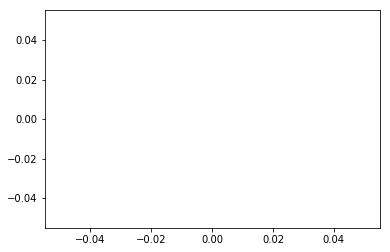In :
HTML(anim.to_html5_video())

Out:

It seems like maybe the morning does better than the afternoon? Let's investigate by looking at the distribution of correlation coefficients of time vs. performance over all the days.

In :
coefs = []
for tup in round_data[day_identifiers].drop_duplicates().values:
year,tourn_num,course_num,round_num = tup
mask = (round_data.Tournament_Year==year) & \
(round_data['Permanent_Tournament_#']==tourn_num) & \
(round_data['Course_#']==course_num) & \
(round_data.Round_Number==round_num)
x = get_seconds(day.Tee_Time).values
y = day.Net_Result.values
coefs.append(np.corrcoef(x,y)[0,1])

In :
plt.hist(coefs,bins=30);
plt.axvline(np.mean(coefs),c='red');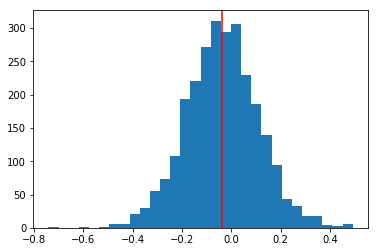Maybe there is a little something there but seems like nothing major. I won't bother looking at this hypothesis formally for now since that is not my goal this time.

Let's now fit a model for every day in the dataset and save the model's predition so we can then subtract it from Net_Result.

In :
## fit model for each day and store the results in the DataFrame
round_data['day_model_pred'] = 0
for tup in round_data[day_identifiers].drop_duplicates().values:
year,tourn_num,course_num,round_num = tup
mask = (round_data.Tournament_Year==year) & \
(round_data['Permanent_Tournament_#']==tourn_num) & \
(round_data['Course_#']==course_num) & \
(round_data.Round_Number==round_num)
x = get_seconds(day.Tee_Time).values
y = day.Net_Result.values
ns = NaturalSpline()
ns.fit(x,y)
y_ = ns.predict(x)

In :
## sanity check that all the predictions were filled
(round_data.day_model_pred==0).mean()

Out:
0.0
In :
## plot a histogram
round_data.day_model_pred.hist(bins=30);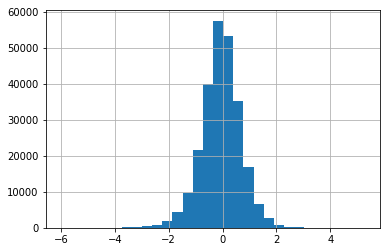In :
## finally, add Net_Result2 - Net_Result adjusted by the intra-day predictions
round_data['Net_Result2'] = round_data.Net_Result - round_data.day_model_pred


Now that we have the Net_Result2 variable - an outcome for each player's round that is adjusted according to their personalized expected performance and the performances of those playing around the same time during the same day - we can compute the same measure of variance within each group that we used in Part 1 - the average pariwise distance between the members of each group - and compare that with the distribution of this metric one would expect according to chance.

In :
## define metric function
def compute_metric(sample):
from scipy.spatial.distance import pdist
return np.mean(pdist(sample[:,None]))

In :
## compute metric by looking at average pairwaise distance within grouping
metrics = []
for tup,group in round_data.groupby(['Tournament_Year',
'Permanent_Tournament_#',
'Course_#','Round_Number',
'Tee_Time','Hole_Started_On']):
metrics.append(compute_metric(group.Net_Result2.values))
metric_unpermuted = np.mean(metrics)
metric_unpermuted

Out:
3.1849814247566361
In :
## extract values and number of twosomes and number of threesomes
result_values = round_data.Net_Result2.values
counts = round_data.groupby(['Tournament_Year',
'Permanent_Tournament_#',
'Course_#','Round_Number',
'Tee_Time','Hole_Started_On']).size()\
.reset_index().rename(columns={0:'Num_Groups'})\
.Num_Groups.value_counts()
num_twos,num_threes = counts,counts

In :
## indicies to split array at (no need for last index)
indicies = np.cumsum(np.concatenate([*num_twos,
*num_threes]))[:-1]

In :
## permute 10,000 times and compute metric each time
metrics_permuted = []
for i in range(10000):
result_values = np.random.permutation(result_values)
samples = np.split(result_values,indicies)
metrics_permuted.append(np.mean([compute_metric(sample) for sample in samples]))

In :
## make fancy plot of results
fig,ax = plt.subplots(1,1,figsize=(16,10))
plt.violinplot(metrics_permuted,vert=False);
plt.axvline(metric_unpermuted,lw=2.3,
label='Value that Occured in Reality',c='red');
plt.xlabel('Average of average pairwise distance within group',
fontsize=20);
plt.ylabel('Density',fontsize=20);
plt.title('''Distribution of Metric Occuring by Chance
Along with Value that Occured in Reality''',fontsize=23);
ax.tick_params(labelsize=16);
plt.legend(loc=2,prop={'size':16});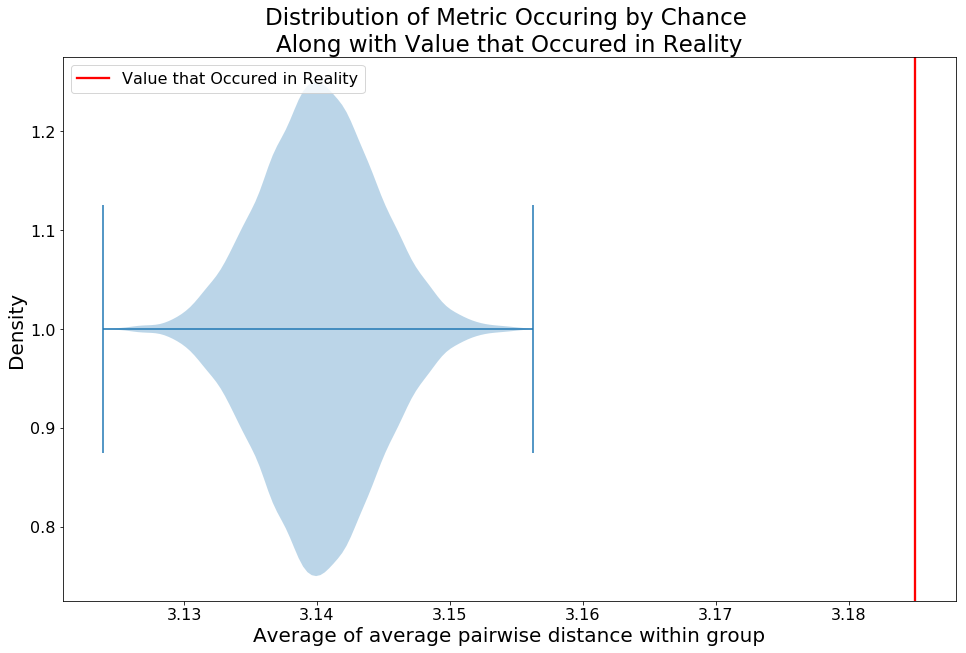So this was unexpected. This is telling us that the metric that occured in reality is actually larger that what you'd expect by chance, in a statistically significant way. This is the exact opposite finding that I found in Part 1.

At first, I have to admit that I was confused by this result. Then I stopped to think about how I was doing the resampling of the data. This result actually made me come to the realization that resampling at the global level on the entire dataset is an invalid approach to what I am trying to get at - whether golfers' playing with each other causes their scores to be more closely bunched together than what you'd expect according to chance.

This strategy is invalid whether you are correcting for the difficult of the course for the day (and changing with time within the day) or not because there are factors that impact all the players playing on the same day, like the difficulty of the conditions. When I resampled at a global level, ignoring the fact that the results were recording on different days, there was a portion of the variance that I observed that was attributable to the difference in difficulty of the course and conditions on the different days. When I compared this distribution to the variance that occured in reality, I obtained the result that the variance within the group was much lower than expected. This effect could have been caused by players in the same group feeding off each other or from something less interesting - the fact that the difficulty of the course and conditions changes from day to day.

When I fit the models above to control for the difficulty of the conditions changing with time within each day, the overall difficulty of the course and conditions of the day where built into that model. Controlling for this decreased the variance in performance within the randomly generated pairings substantially.

To test truly test my hypothesis, I need to do the resamping within each day to see whether the variance within the pairings is less than what you'd expect according to random chance. I will do this with one day first and then I will do it for each day.

In :
## the day currently in memory

Out:
Tournament_Year Permanent_Tournament_# Course_# Player_Number Player_Name Round_Number Event_Name Course_Name Tee_Time Round_Score End_of_Round_Finish_Pos._(text) Hole_Started_On Field_Ave Strokes_Gained Exp_StrokesGained Net_Result day_model_pred
252745 2016 60 688 1810 Mickelson, Phil 4 TOUR Championship East Lake GC 11:55 66 22 1.0 68.0 2.0 1.069470 0.930530 0.0
252746 2016 60 688 23108 Kuchar, Matt 4 TOUR Championship East Lake GC 13:05 69 T15 1.0 68.0 -1.0 1.021468 -2.021468 0.0
252747 2016 60 688 24140 O'Hair, Sean 4 TOUR Championship East Lake GC 11:45 65 T17 1.0 68.0 3.0 0.110816 2.889184 0.0
In :
## return to just one day's worth of data
day = round_data[(round_data.Tournament_Year==2003) &
(round_data['Permanent_Tournament_#']==1) &
(round_data['Course_#']==101) &
(round_data.Round_Number==3)]

In :
## same calculation as above but for the one day
metrics = []
for tup,group in day.groupby(['Tee_Time','Hole_Started_On']):
metrics.append(compute_metric(group.Net_Result2.values))
metric_unpermuted = np.mean(metrics)
metric_unpermuted

result_values = day.Net_Result2.values
counts = day.groupby(['Tee_Time','Hole_Started_On']).size()\
.reset_index().rename(columns={0:'Num_Groups'})\
.Num_Groups.value_counts()
num_twos,num_threes = counts,counts

indicies = np.cumsum(np.concatenate([*num_twos,
*num_threes]))[:-1]

metrics_permuted = []
for i in range(1000):
result_values = np.random.permutation(result_values)
samples = np.split(result_values,indicies)
metrics_permuted.append(np.mean([compute_metric(sample) for sample in samples]))

fig,ax = plt.subplots(1,1,figsize=(16,10))
plt.violinplot(metrics_permuted,vert=False);
plt.axvline(metric_unpermuted,lw=2.3,
label='Value that Occured in Reality',c='red');
plt.xlabel('Average of average pairwise distance within group',
fontsize=20);
plt.ylabel('Density',fontsize=20);
plt.title('''Distribution of Metric Occuring by Chance
Along with Value that Occured in Reality''',fontsize=23);
ax.tick_params(labelsize=16);
plt.legend(loc=2,prop={'size':16});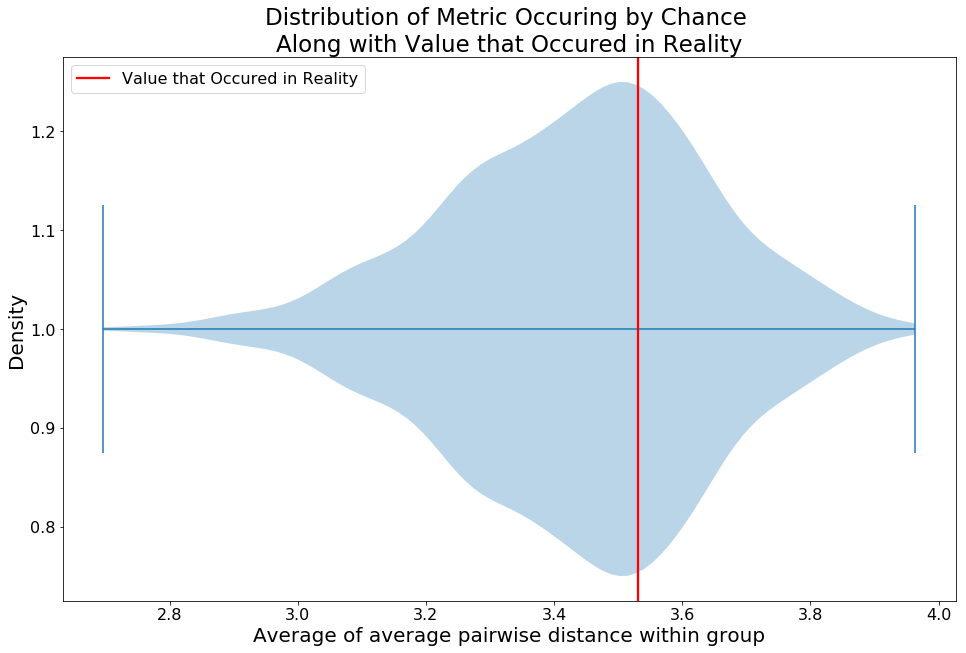This result says that the variance within the groups is about what you should expect according to random chance. Let's do this calculation for every day. I will store the percentile of where each value that occured in reality falls in the distribution of the metrics calculated for each resampling for each day.

In :
## resample inside each day's results
from scipy.stats import percentileofscore
day_identifiers = ['Tournament_Year','Permanent_Tournament_#',
'Course_#','Round_Number']
round_data['day_model_pred'] = 0
percentiles = []
for tup in round_data[day_identifiers].drop_duplicates().values:
year,tourn_num,course_num,round_num = tup
mask = (round_data.Tournament_Year==year) & \
(round_data['Permanent_Tournament_#']==tourn_num) & \
(round_data['Course_#']==course_num) & \
(round_data.Round_Number==round_num)

metrics = []
for tup,group in day.groupby(['Tee_Time','Hole_Started_On']):
metrics.append(compute_metric(group.Net_Result2.values))
metric_unpermuted = np.mean(metrics)

result_values = day.Net_Result2.values
counts = day.groupby(['Tee_Time','Hole_Started_On']).size()\
.reset_index()\
.rename(columns={0:'Num_Groups'})\
.Num_Groups.value_counts()
num_twos,num_threes = (counts if 2 in counts else 0,
counts if 3 in counts else 0)

indicies = np.cumsum(np.concatenate([*num_twos,
*num_threes]))[:-1]\
.astype(int)

metrics_permuted = []
for i in range(1000): ## run 1000 this time in interest of time
result_values = np.random.permutation(result_values)
samples = np.split(result_values,indicies)
metrics_permuted.append(np.mean([compute_metric(sample)
for sample in samples]))

percentiles.append(percentileofscore(metrics_permuted,
metric_unpermuted))

In :
## make a histogram of the percentiles of each day's intra-group
## variance within the distribution that would happen by chance
plt.hist(percentiles);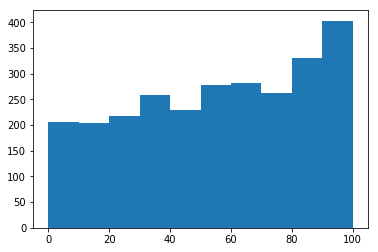So, it turns out that I was wrong! Seems like there is even weak evidence that the amount of variance within the groupings is higher than you'd expect from chance. So I will not bother to answer the further questions posed in the beginning because it seems like the effect I was hypothesizing doesn't exist after all!

From this post, I have the following take aways:

• Inference is a much more challenging task than prediction
• Revisiting the assumptions of an analysis after some time has passed can be a humbling experience.
Written on May 3, 2018